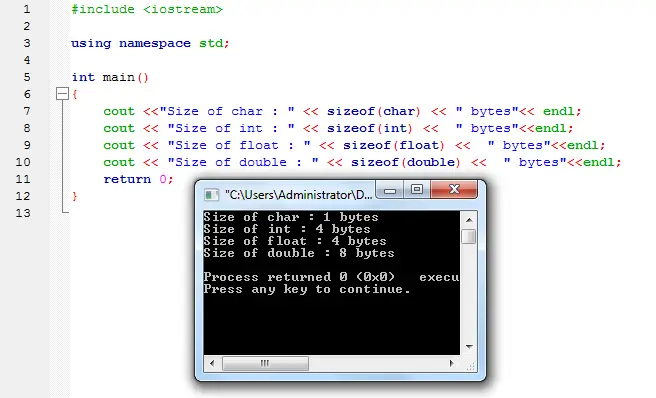# C++ sizeof Operator• Version
• File Size 1.30 KB
• File Count 1
• Create Date May 9, 2016
• Last Updated May 9, 2016

# C++ sizeof Operator

C++ sizeof Operator
sizeof Operator will return a number of bytes in a variable or data type. This c++ program will display the size of 4 datatypes (char, int, float and double) in bytes.

Open the main.cpp in codeblocks or whatever ide you are using that supports c++.

Source code:

```#include < iostream >

using namespace std;

int main()
{
cout << "Size of char : " <<  sizeof(char) << " bytes" <<  endl;
cout << "Size of int : " <<  sizeof(int) <<  " bytes"  << endl;
cout << "Size of float : " <<  sizeof(float) <<  " bytes" << endl;
cout << "Size of double : " << sizeof(double) <<  " bytes" << endl;
return 0;
}

```